SSC CGL Previous Year Questions: Geometry- 3

# SSC CGL Previous Year Questions: Geometry- 3 - SSC CGL

Test Description

## 40 Questions MCQ Test SSC CGL (Tier - 1) - Previous Year Papers (Topic Wise) - SSC CGL Previous Year Questions: Geometry- 3

SSC CGL Previous Year Questions: Geometry- 3 for SSC CGL 2023 is part of SSC CGL (Tier - 1) - Previous Year Papers (Topic Wise) preparation. The SSC CGL Previous Year Questions: Geometry- 3 questions and answers have been prepared according to the SSC CGL exam syllabus.The SSC CGL Previous Year Questions: Geometry- 3 MCQs are made for SSC CGL 2023 Exam. Find important definitions, questions, notes, meanings, examples, exercises, MCQs and online tests for SSC CGL Previous Year Questions: Geometry- 3 below.
Solutions of SSC CGL Previous Year Questions: Geometry- 3 questions in English are available as part of our SSC CGL (Tier - 1) - Previous Year Papers (Topic Wise) for SSC CGL & SSC CGL Previous Year Questions: Geometry- 3 solutions in Hindi for SSC CGL (Tier - 1) - Previous Year Papers (Topic Wise) course. Download more important topics, notes, lectures and mock test series for SSC CGL Exam by signing up for free. Attempt SSC CGL Previous Year Questions: Geometry- 3 | 40 questions in 40 minutes | Mock test for SSC CGL preparation | Free important questions MCQ to study SSC CGL (Tier - 1) - Previous Year Papers (Topic Wise) for SSC CGL Exam | Download free PDF with solutions
 1 Crore+ students have signed up on EduRev. Have you?
SSC CGL Previous Year Questions: Geometry- 3 - Question 1

### O is the centre of a circle and AB is the tangent to it touching at B. If OB = 3 cm. and OA = 5 cm, then the measure of AB in cm is    (SSC CGL 1st Sit. 2016)

Detailed Solution for SSC CGL Previous Year Questions: Geometry- 3 - Question 1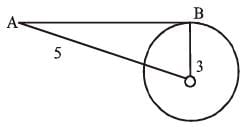OA2 = OB2 + BA2
AB2 = 52 – 32
= 25 – 9 = 16
∴ AB = 4

SSC CGL Previous Year Questions: Geometry- 3 - Question 2

### In a ΔABC, BC is extended upto D: ∠ACD = 120°, ∠B = 1/2∠A. Then ∠A is   (SSC CGL 1st Sit. 2016)

Detailed Solution for SSC CGL Previous Year Questions: Geometry- 3 - Question 2

∠A + ∠B = ∠ACD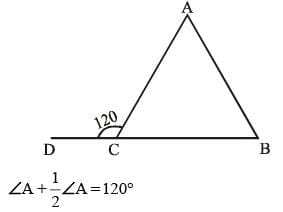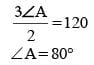SSC CGL Previous Year Questions: Geometry- 3 - Question 3

### BE and CF are two altitudes of a triangle ABC. If AB = 6 cm, AC = 5 cm and CF = 4 cm, then the length of BE is  (SSC CGL 1st Sit. 2016)

Detailed Solution for SSC CGL Previous Year Questions: Geometry- 3 - Question 3

From question, A B = 6 cm, AC = 5 cm, CF = 4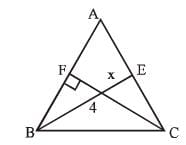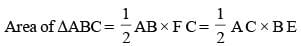6 × 4 = 5 × x {where BE = x}
24/5 = x
x = 4.8 cm.

SSC CGL Previous Year Questions: Geometry- 3 - Question 4

O is the orthocentre of ΔABC , and if ∠BOC = 110° then ∠BAC will be   (SSC CGL 1st Sit. 2016)

Detailed Solution for SSC CGL Previous Year Questions: Geometry- 3 - Question 4

For Orthocentre ∠BAC = 180 – ∠BOC
= 180 – 110 = 70°

SSC CGL Previous Year Questions: Geometry- 3 - Question 5

If D, E and F are the mid points  of BC, CA and AB respectively of the ΔABC then the ratio of area of the parallelogram DEFB and area of the trapezium CAFD is:   (SSC CGL 1st Sit. 2015)

Detailed Solution for SSC CGL Previous Year Questions: Geometry- 3 - Question 5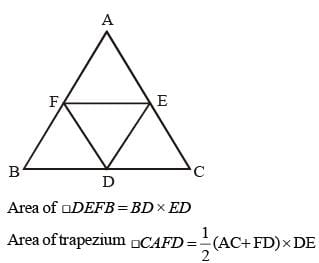Here  AC = 2 AE = 2FD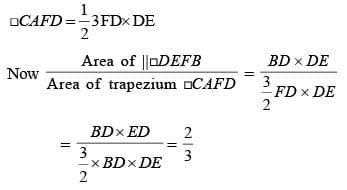SSC CGL Previous Year Questions: Geometry- 3 - Question 6

If a person travels from a point L towards east for 12 km and then travels 5 km towards north and reaches a point M, then shortest distance from L to M is:    (SSC CGL 1st Sit. 2015)

Detailed Solution for SSC CGL Previous Year Questions: Geometry- 3 - Question 6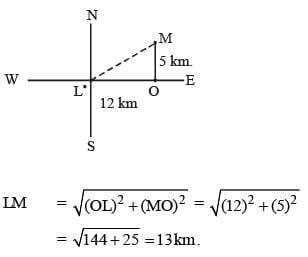SSC CGL Previous Year Questions: Geometry- 3 - Question 7

G is the centroid of ΔABC. The medians AD and BE intersect at right angles. If the lengths of AD and BE are 9 cm and 12 cm respectively; then the length of AB (in cm) is?   (SSC CGL 1st Sit. 2015)

Detailed Solution for SSC CGL Previous Year Questions: Geometry- 3 - Question 7

BE = 12 cm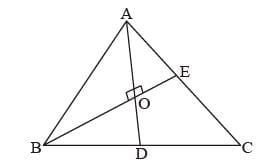∴ ∠AOB = 90°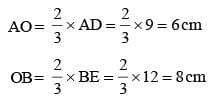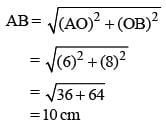SSC CGL Previous Year Questions: Geometry- 3 - Question 8

If the measure of three angles of a triangle are in the ratio 2 : 3 : 5, then the triangle is:    (SSC CGL 1st Sit. 2015)

Detailed Solution for SSC CGL Previous Year Questions: Geometry- 3 - Question 8

Sum of the angle of a triangle = 180°
⇒ 2x° + 3x° + 5x° = 180°
⇒ 10x° = 180°
x° = 18°
Angle are = 36°, 54°, 90° So, this is right angles triangle.

SSC CGL Previous Year Questions: Geometry- 3 - Question 9

Internal bisectors of ∠Q and ∠R of ΔPQR intersect at O. If ∠ROQ = 96° then the value of ∠RPQ is:   (SSC CGL 1st Sit. 2015)

Detailed Solution for SSC CGL Previous Year Questions: Geometry- 3 - Question 9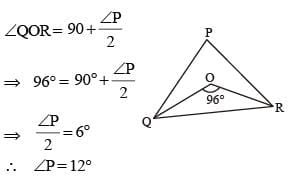SSC CGL Previous Year Questions: Geometry- 3 - Question 10

If the altitude of an equilateral triangle is 12√3 cm, then its area would be:    (SSC CGL 1st Sit. 2015)

Detailed Solution for SSC CGL Previous Year Questions: Geometry- 3 - Question 10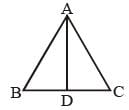Let ΔABC is a equilateral triangle with AD as an altitude from A on side BC.
Let AB = BC = AC = x
From question AD = 12√3 cm.
then from ΔABD,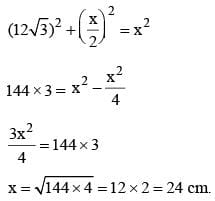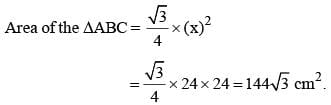SSC CGL Previous Year Questions: Geometry- 3 - Question 11

If the number of vertices, edges and faces of a rectangular parallelopiped are denoted by v, e and f respectively, the value of (v – e + f) is   (SSC CGL 1st Sit. 2015)

Detailed Solution for SSC CGL Previous Year Questions: Geometry- 3 - Question 11

The value of = v – e + f
= 8 – 12 + 6 = 2.

SSC CGL Previous Year Questions: Geometry- 3 - Question 12

If the three angles of a triangle are: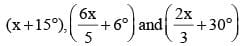then the triangle is:   (SSC CGL 1st Sit. 2015)

Detailed Solution for SSC CGL Previous Year Questions: Geometry- 3 - Question 12

Angles are = (x + 15°),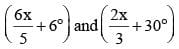We know that
Sum of the angles of a triangle is 180°.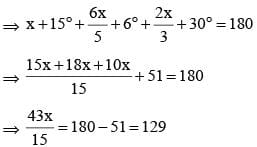⇒ 43x = 129 x 15
x = 45°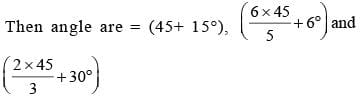= 60°, 60°, 60°
So this is an equilateral triangle.

SSC CGL Previous Year Questions: Geometry- 3 - Question 13

Let C1 and C2 be the inscribed and circumscribed circles of a triangle with sides 3 cm, 4 cm and 5 cm then area of C1 to area of C2 is    (SSC CGL 1st Sit. 2015)

Detailed Solution for SSC CGL Previous Year Questions: Geometry- 3 - Question 13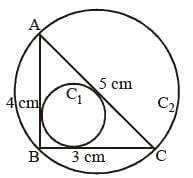Let ΔABC has three sides BC, AB and AC equal to 3 cm, 4 cm and 5 cm respectively.
Now, as, (5)2 = (3)2 + (4)2 i.e. (AC)2 = (AB)2 + (BC)2
∴ ΔABC is a right angle triangle
Then, for circumcircle C2, radius = AC/2 = 5/2 = 2.5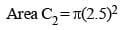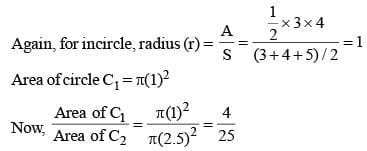SSC CGL Previous Year Questions: Geometry- 3 - Question 14

If a clock started at noon, then the angle turned by hour hand at 3.45 PM is    (SSC CGL 1st Sit. 2015)

Detailed Solution for SSC CGL Previous Year Questions: Geometry- 3 - Question 14

Clock started at 12 pm
Angle turned by hour hand in one hour = 360/12 = 30°
Angle turned by hour hand in one minute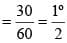Angle turned  by hour hand in 3 hour 45 minutes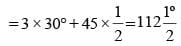SSC CGL Previous Year Questions: Geometry- 3 - Question 15

In a parallelogram PQRS, angle P is four times of angle Q, then the measure of ∠R is     (SSC CGL 1st Sit. 2015)

Detailed Solution for SSC CGL Previous Year Questions: Geometry- 3 - Question 15

P = 4Q
P + Q = 180°
4Q + Q = 180°
∵ 180/5 = 36°
So, R = 180° –  36° = 144°

SSC CGL Previous Year Questions: Geometry- 3 - Question 16

Two chords of length a unit and b unit of a circle make angles 60° and 90° at the centre of a circle respectively, then the correct relation is (SSC CGL 1st Sit. 2015)

Detailed Solution for SSC CGL Previous Year Questions: Geometry- 3 - Question 16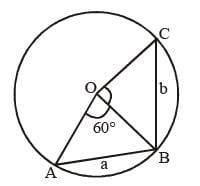Let the chord AB = a and chord BC = b makes angle
∠ AOB = 60° and ∠ BOC = 90° at the center ‘O’ of the circle. There, OA = OB = OC = radius
In ΔAOB, ∠ OAB = ∠ OBA
and ∠ AOB = 60°
∴ ∠ OAB + ∠ OBA = 180° – 60° = 120°
⇒ ∠ OAB + ∠ OAB = 120°
⇒ ∠ OAB = 60°
Thus, ∠ OAB = ∠ OBA = ∠ AOB = 60°
∴ AOB is equilateral triangle
Hence, AO = OB = AB = a unit
Now, from ΔBOC, ∠ BOC = 90°, BC = b unit
OB = OC = a unit
(BC)2 = (OB)2 + (OC)2
b2 = a2 + a2 ⇒ b2 = 2a2
b = √2 a

SSC CGL Previous Year Questions: Geometry- 3 - Question 17

The sides of a triangle having area 7776 sq. cm are in the ratio 3 : 4 : 5. The perimeter of the triangle is     (SSC CGL 1st Sit. 2015)

Detailed Solution for SSC CGL Previous Year Questions: Geometry- 3 - Question 17

Let sides of Δ be 3x, 4x, 5x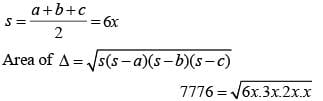7776 = 6x2
∴ x = 36
Sides of Δ will be 108, 144 and 180
Perimeter of Δ is 108 +144 + 180 = 432 cm

SSC CGL Previous Year Questions: Geometry- 3 - Question 18

The measure of an angle whose supplement is three times as large as its complement, is    (SSC CGL 1st Sit. 2015)

Detailed Solution for SSC CGL Previous Year Questions: Geometry- 3 - Question 18

Let ‘x’ be the measure of an angle.
Then its complement angle = 90° – x
and its supplement angle = 180° – x
According to question
(180° – x) = 3(90° – x)
180° – x = 270° – 3x
2x = 90°
x = 45°

SSC CGL Previous Year Questions: Geometry- 3 - Question 19

Two poles of height 7 m and 12 m stand on a plane ground. If the distance between their feet is 12 m, the distance between their top will be    (SSC CGL 1st Sit. 2015)

Detailed Solution for SSC CGL Previous Year Questions: Geometry- 3 - Question 19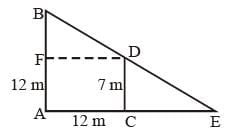Let AB ad CD are two poles of height 12 m and 7 m separated by a distance AC = 12 m
Draw a line DF || AC
Then DF = 12 m
and BF = AB – AF = AB – CD = 12 – 7 = 5m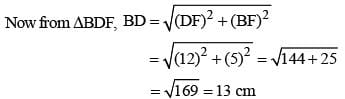∴ Distance between the top of two poles BD = 13 m.

SSC CGL Previous Year Questions: Geometry- 3 - Question 20

A tangent is drawn to a circle of radius 6cm from a point situated at a distance of 10 cm from the centre of the circle.
The length of the tangent will be    (SSC CGL 1st Sit. 2015)

Detailed Solution for SSC CGL Previous Year Questions: Geometry- 3 - Question 20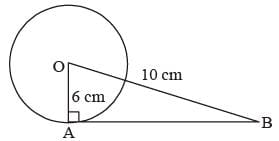AB2 + OA2 = OB2  ⇒ AB2 = OB2 = OA2
AB2 = (10)2 – (6)2 = 100 – 36 = 64
AB = 8cm

SSC CGL Previous Year Questions: Geometry- 3 - Question 21

In ΔABC, a line through A cuts the side BC at D such that BD : DC = 4 : 5. If the area of ΔABD = 60 cm2, then the area of ΔADC is    (SSC CGL 1st Sit. 2015)

Detailed Solution for SSC CGL Previous Year Questions: Geometry- 3 - Question 21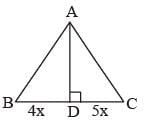Let AE is the height of the triangle ABC, then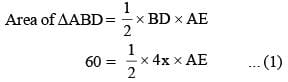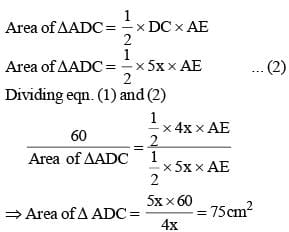SSC CGL Previous Year Questions: Geometry- 3 - Question 22

Two circles of radii 5 cm and 3 cm touch externally, then the ratio in which the direct common tangent to the circles divides externally the line joining the centres of the circles is:    (SSC CHSL 2015)

Detailed Solution for SSC CGL Previous Year Questions: Geometry- 3 - Question 22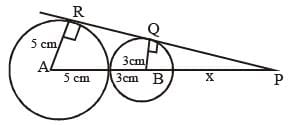Let PB = x cm.
In ∠PQB and PRA,
∠Q = ∠R = 90°, ∠P = ∠P  {common}
∴ ΔPQB ~ ΔPRA   {AA criteria}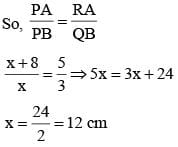Now, point P divide the line joining the centers of two circles externally into.
AP : PB = (8 + x) : x
= (8 + 12) : 12 = 20 : 12 ⇒ 5 : 3

SSC CGL Previous Year Questions: Geometry- 3 - Question 23

In ΔABC, AB = BC = K, AC = √2 K, then ΔABC is a:    (SSC CHSL 2015)

Detailed Solution for SSC CGL Previous Year Questions: Geometry- 3 - Question 23

In ΔABC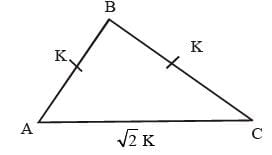AC = 2K
AC2 = 2K2
AC2 = AB2 + BC2
So ΔABC is right angled triangle
So, in ΔABC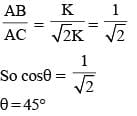So, In triangle ABC, ∠B = 90°; ∠C =45°; ∠A = 45°
Hence, triangle ABC is right isoscles triangle.

SSC CGL Previous Year Questions: Geometry- 3 - Question 24

The distance between centres of two circles of radii 3 cm and 8 cm is 13 cm. If the points of contact of a direct common tangent to the circles are P and Q, then the length of the lien segment PQ is:    (SSC CHSL 2015)

Detailed Solution for SSC CGL Previous Year Questions: Geometry- 3 - Question 24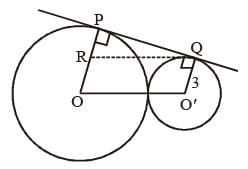Draw a line RQ || OO'
then O' Q = RO = 3 cm
Now PR = OP – RO
= 8 – 3 = 5 cm
From ΔPQR, RQ = OO' = 13 cm {given}
(PQ)2 = (RQ)2 – (PR)2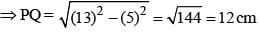SSC CGL Previous Year Questions: Geometry- 3 - Question 25

ABCD is a square. Draw a triangle QBC on side BC considering BC as base and draw a triangle PAC on AC as its base such that Δ QBC ~ Δ PAC.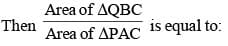(SSC CHSL 2015)

Detailed Solution for SSC CGL Previous Year Questions: Geometry- 3 - Question 25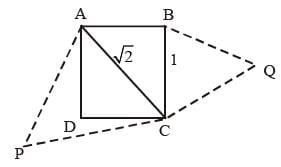ABCD is a square.
Δ QBC ~ Δ PAC  (Given)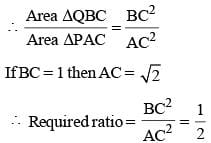SSC CGL Previous Year Questions: Geometry- 3 - Question 26

In ΔABC, ∠B = 60°, and ∠C = 40°; AD and AE are respectively the bisector of ∠A and perpendicular on BC. The measure of ∠EAD is:    (SSC CHSL 2015)

Detailed Solution for SSC CGL Previous Year Questions: Geometry- 3 - Question 26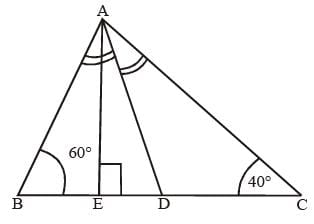In ΔABC
∠A = 180°- (60° + 40°) = 80°
In ∠AEC
∠EAC = 180° – (90° + 40°) = 50°
= 50° – 40°

SSC CGL Previous Year Questions: Geometry- 3 - Question 27

The diagonal of a quadrilateral shaped field is 24m an d the perpendiculars dropped on it from the remaining opposite vertices are 8m and 13m. The area of the field is:    (SSC Sub. Ins. 2015)

Detailed Solution for SSC CGL Previous Year Questions: Geometry- 3 - Question 27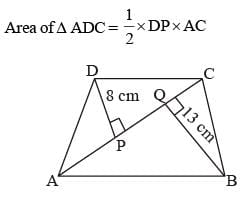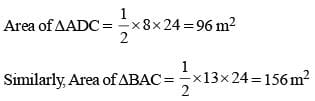Area of Quadrilateral = 96 + 156 = 252 m2

SSC CGL Previous Year Questions: Geometry- 3 - Question 28

The perimeters of two similar triangles are 30 cm and 20cm respectively. If one side of the first triangle is 9cm. Determine the corresponding side of the second triangle:    (SSC Sub. Ins. 2015)

Detailed Solution for SSC CGL Previous Year Questions: Geometry- 3 - Question 28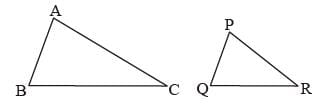Let ΔABC and ΔPQR are two similar triangles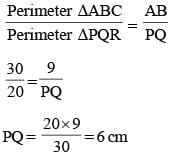SSC CGL Previous Year Questions: Geometry- 3 - Question 29

Two isosceles triangles have equal vertical angles and their areas are in the ratio 9 : 16. Then the ratio of their corresponding heights is:   (SSC Sub. Ins. 2015)

Detailed Solution for SSC CGL Previous Year Questions: Geometry- 3 - Question 29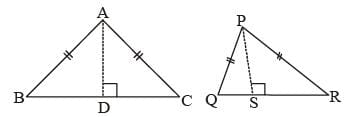Let ΔABC and ΔPQR are two isosceles triangles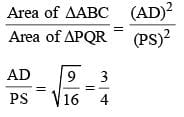SSC CGL Previous Year Questions: Geometry- 3 - Question 30

Two circles of radii 10 cm and 8 cm intersect and the length of the common chord is 12 cm. Then the distance between their centres is:   (SSC Sub. Ins. 2015)

Detailed Solution for SSC CGL Previous Year Questions: Geometry- 3 - Question 30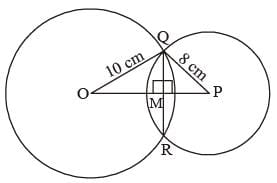Line joining the centre is ⊥ bisector of common chord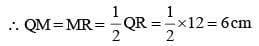In ΔOMQ, ∠OMQ = 90°
OQ2 = OM2 + MQ2 (Pythagorus theorem)
102 = OM2 + 62
OM2 = 100 – 36 = 64
OM = 8cm
In ΔQMP, ∠QMP = 90°
QP2 = QM2 + PM2 (Pythagorus theorem)
82 = 62 + PM2
PM = 64 – 36 = √28 = 2√7
OP = OM + MP = 8 + 2√7
So distance between centres O and P
= 8 + 2√7= 13.3 cm

SSC CGL Previous Year Questions: Geometry- 3 - Question 31

If in a triangle ABC,  BE and CF are two medians perpendicular to each other and if AB = 19cm and AC = 22cm then the length of BC is:    (SSC Sub. Ins. 2015)

Detailed Solution for SSC CGL Previous Year Questions: Geometry- 3 - Question 31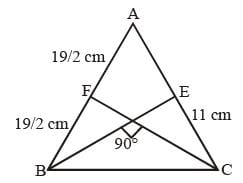From question, median BE ⊥ CF.
In this case we know that
(AB)2 + (AC)2 = 5 (BC)2
(19)2 + (22)2 = 5 (BC)2
361 + 484 = 5 (BC)2
845 = 5 (BC)2
BC = √169 = 13 cm

SSC CGL Previous Year Questions: Geometry- 3 - Question 32

If two circles of radii 9 cm and 4 cm touch externally, then the length of a common tangent is   (SSC CGL 1st Sit. 2014)

Detailed Solution for SSC CGL Previous Year Questions: Geometry- 3 - Question 32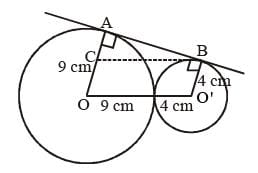In figure, AC = AO – CO
= 9 cm – 4 cm  = 5 cm {∵ CO = BO'}
Also, CB = OO' = 13 cm
In ΔABC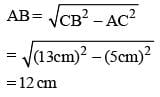SSC CGL Previous Year Questions: Geometry- 3 - Question 33

The interior angle of a regular polygon is 140°. The number of sides of that polygon is    (SSC CGL 1st Sit. 2014)

Detailed Solution for SSC CGL Previous Year Questions: Geometry- 3 - Question 33

Let n be the number of sides.
(n – 2) × 180° = 140° × n
180n – 360 = 140 n
40n = 360
n = 360/40 = 9

SSC CGL Previous Year Questions: Geometry- 3 - Question 34

Two parallel chords of a circle of diameter 20 cm are 12 cm and 16 cm long. If the chords are in the same side of the centre, then the distance between them is    (SSC CGL 1st Sit. 2014)

Detailed Solution for SSC CGL Previous Year Questions: Geometry- 3 - Question 34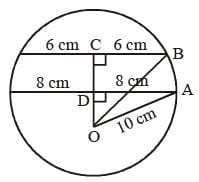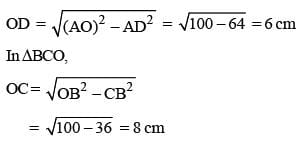distance between chords = OC – OD
= 2CM

SSC CGL Previous Year Questions: Geometry- 3 - Question 35

If O is the in-centre of ΔABC; if ∠BOC = 120°, then the measure of ∠BAC is    (SSC CGL 1st Sit. 2014)

Detailed Solution for SSC CGL Previous Year Questions: Geometry- 3 - Question 35While ∠BOC is in circle then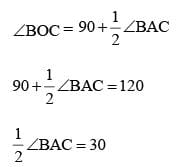∠BAC = 60°

SSC CGL Previous Year Questions: Geometry- 3 - Question 36

In  ΔABC, ∠A < ∠B.  The altitude to the base divides vertex angle C into two parts C1 and C2, with C2 adjacent to BC.
Then    (SSC CGL 1st Sit. 2014)

Detailed Solution for SSC CGL Previous Year Questions: Geometry- 3 - Question 36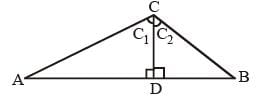In ΔADC, sum of angles = 180°
A + D + C1 = 180°; A + C1 = 180° – 90° = 90°
Similarly,
In ΔBDC, B + D + C2 = 180°; B + C2 = 180° – 90° = 90°
A + C1 = B + C2
C1 – C2 = B – A

SSC CGL Previous Year Questions: Geometry- 3 - Question 37

In a circle with centre O, AB is a chord, and AP is a tangent to the circle. If ∠AOB = 140°, then the measure of  ∠PAB is    (SSC CGL 1st Sit. 2014)

Detailed Solution for SSC CGL Previous Year Questions: Geometry- 3 - Question 37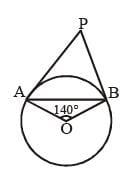In ΔAOB, ∠A + ∠B + ∠O = 180°
∠A + ∠B = 180 – 140° = 40°
∠A = ∠B = 20° {∵ AO = BO = radius}
∴ ∠PAO = 90° {As PA is tangent to the circle}
⇒ ∠PAB + ∠BAO = 90°
∠PAB = 90° – 20° = 70°

SSC CGL Previous Year Questions: Geometry- 3 - Question 38

In ΔABC, E and D are points on sides AB and AC respectively such that ∠ABC = ∠ADE. If AE = 3 cm, AD = 2 cm and EB = 2 cm, then length of DC is    (SSC CGL 1st Sit. 2014)

Detailed Solution for SSC CGL Previous Year Questions: Geometry- 3 - Question 38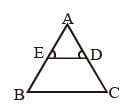∠A = ∠D
∠B = ∠D
Then third angle ∠C = ∠E
(By AAA)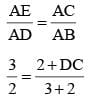15 = 4 + 2 DC
11 = 2 DC
DC = 5.5 cm

SSC CGL Previous Year Questions: Geometry- 3 - Question 39

In a quadrilateral ABCD, the bisectors of ∠A and ∠B meet at O. If ∠C = 70° and ∠D = 130°, then measure of ∠AOB is   (SSC CGL 1st Sit. 2014)

Detailed Solution for SSC CGL Previous Year Questions: Geometry- 3 - Question 39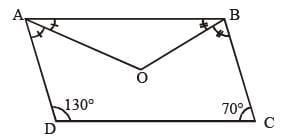A + B + C + D = 360
A + B = 360 – (130 + 70) = 160°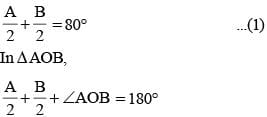∠ AOB = 180° – 80°  = 100°

SSC CGL Previous Year Questions: Geometry- 3 - Question 40

Two circles intersect each other at the points A and B. A straight line parallel to AB intersects the circles at C, D, E and F. If CD = 4.5 cm, then the measure of EF is    (SSC CHSL 2014)

Detailed Solution for SSC CGL Previous Year Questions: Geometry- 3 - Question 40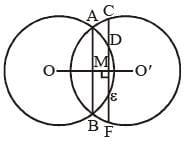As we see, DE is the chord of the circle with center ‘O’
then, line OO' ⊥ bisect DE
∴ DM = ME ...(i)
Again, CF is the chord of the circle with center O'.
Again line OO' ⊥ bisect CF
∴ CM = MF
⇒ CD + DM = ME + EF
4.5 + DM = DM + EF {from (i)}
∴ EF = 4.5 cm

## SSC CGL (Tier - 1) - Previous Year Papers (Topic Wise)

250 tests
Information about SSC CGL Previous Year Questions: Geometry- 3 Page
In this test you can find the Exam questions for SSC CGL Previous Year Questions: Geometry- 3 solved & explained in the simplest way possible. Besides giving Questions and answers for SSC CGL Previous Year Questions: Geometry- 3, EduRev gives you an ample number of Online tests for practice

250 tests